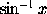User Name Remember Me? Password

 Algebra Pre-Algebra and Basic Algebra Math Forum

 January 11th, 2007, 11:24 AM #11 Senior Member   Joined: Nov 2006 From: I'm a figment of my own imagination :? Posts: 848 Thanks: 0 We know that sin(x)=3/5 Since sin²(x) + cos²(x) = 1, cos²(x) = 1 - 9/25 = (25-9)/25 = 16/25. Therefore, cos(x) = ±4/5. Since the sin^(-1)(3/5) is in the first quadrant, the cosine must also be positive, and we have cos(x) = 4/5 as an exact answer, and, as a bonus, no calculator was used.January 13th, 2007, 03:42 AM #12 Global Moderator   Joined: Dec 2006 Posts: 20,931 Thanks: 2205 In an equation such as w =is not a power of sin(x); it's a notation for a function called the inverse sine (or invsin or arcsin) of x. The inverse sine of x is the acute angle whose sine is x, so the equation above implies that x = sin(w). If x is negative, its inverse sine is negative. Tell me, hatcher777, do you have a textbook which explains the basics of trigonometry (and also mathematical notation in general), including what I've just explained? I ask because your main problem seems to be that you are completely unfamiliar with some of the concepts. I am willing to explain things here, but it will take time. You could proceed much faster using a good introductory textbook.January 13th, 2007, 03:43 AM #13 Global Moderator   Joined: Dec 2006 Posts: 20,931 Thanks: 2205 The inverse tangent works rather like inverse sine, but the inverse cosine is a bit different because cosine is an even function, whereas sine and tangent are odd functions.Tags exact, valuesSearch tags for this page
,

,

,

,

,

,

,

,

,

,

,

,

,

,

# exact value of cos(4pi/5)

Click on a term to search for related topics.
 Thread ToolsShow Printable VersionEmail this Page Display ModesLinear ModeSwitch to Hybrid ModeSwitch to Threaded ModeSimilar Threads Thread Thread Starter Forum Replies Last Post choi2463 Trigonometry 3 May 7th, 2013 06:32 PM MRKhal Algebra 2 March 4th, 2011 07:13 PM space55 Calculus 0 October 10th, 2010 03:23 PM mtt0216 Algebra 16 March 8th, 2010 04:09 PM gretchen Algebra 1 April 1st, 2007 01:01 AM

 Contact - Home - Forums - Cryptocurrency Forum - Top

Copyright © 2019 My Math Forum. All rights reserved.